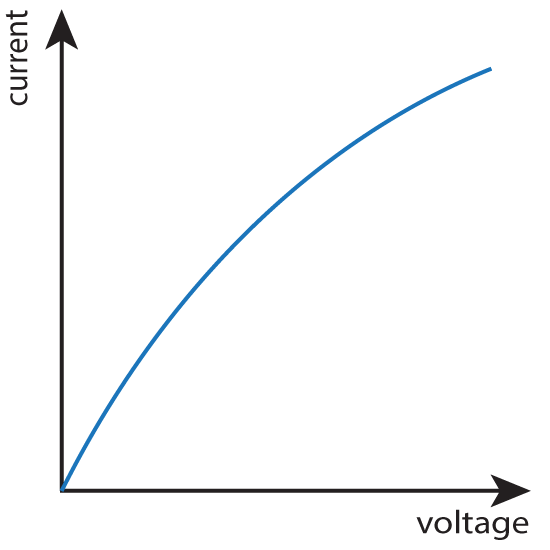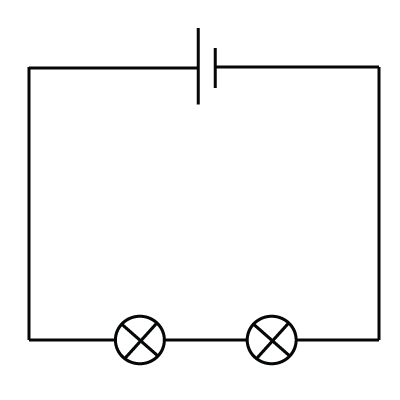1-4. What are the missing words in this paragraph describing the nature of electricity?

 The flow of electricity round a circuit is called the ? current voltage charge resistance energy power . The 'kick' given by the battery is the ? current voltage charge resistance energy power . Tiny ? atoms nuclei charges molecules are actually flowing through the wires, and in metallic conductors these are ? electrons protons neutrons atoms nuclei molecules .
5+6:Which components have the following characteristic graphs - showing how the current varies as the voltage is changed?
5.6.7. Which component is shown here?8.Which of these answers gives the correct way of connecting each meter?

 Ammeter Voltmeter A) Series Series B) Parallel Parallel C) Series Parallel D) Parallel Series

9+10: A student is experimenting with identical bulbs and 6V cells.

The bulb in this circuit has a current of 2A passing through it when connected to a single 6V cell as shown.What are the approximate values for the currents in the following circuits using the same bulbs and cells?

9.10.11+12:The table below shows measurements taken on a selection of resistors. What are the missing values?

 Voltage (V) Current (A) Resistance (Ω) 11. 60 ? 30 120000 33.33 0.03 2000 12. ? 30 120 300 0.0083 0.5 60